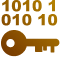# Decimal to Fraction - Fraction to Decimal

## Convert Decimal to Fraction, Decimal to Mixed Fraction. Convert Fraction to Decimal, Mixed Fraction to Decimal

Home

Converter

Decimal Fraction Converter

You can input in any of the fields and get equivalent values.
=
=

## Level Up Your Math Skills with Decimal to Fraction and Fraction to Decimal Conversion• Decimals and fractions are two ways of representing numbers.
• Decimals use a decimal point to separate the whole number part of a number from the fractional part. Fractions use a fraction bar to separate the numerator (the number of parts) from the denominator (the total number of parts).
• The decimal system is a base-10 number system, meaning that the position of each digit in a number represents a power of 10. The fraction system is a base-60 number system, meaning that the position of each digit in a fraction represents a power of 60.
• Decimals can be converted to fractions by multiplying the decimal by 10, 100, 1000, and so on, until the fractional part ends with a zero. This will give us a denominator with a number of nines equal to the number of digits after the decimal point. We can then simplify the fraction by dividing the numerator and denominator by the greatest common factor.
• Fractions can be converted to decimals by dividing the numerator by the denominator. If the numerator is not divisible by the denominator, we can continue to divide, bringing down zeros in the decimal part until we reach a repeating pattern or the denominator goes into the numerator with no remainder. If there is a repeating pattern in the decimal part, we can convert the fraction to a decimal by subtracting the non-repeating part from the repeating part and dividing by the length of the repeating part.
• Decimals and fractions can be used to represent the same number. For example, 0.5 is equal to 1/2. Decimals and fractions are both useful ways to represent numbers, and the best way to represent a number will depend on the context.
Here are some examples of decimals and fractions in the real world:
• Decimals:
• Money: We use decimals to represent money, such as \$5.25.
• Measurements: We use decimals to represent measurements, such as 3.25 meters or 12.5 ounces.
• Percentages: We use decimals to represent percentages, such as 22% or 75%.
• Fractions:
• Recipes: We use fractions to represent ingredients in recipes, such as 1/2 cup of flour or 1/4 cup of milk.
• Time: We use fractions to represent time, such as 1/2 hour or 3/4 of a day.
• Sports: We use fractions to represent scores and stats in sports, such as 1/2 time, or 3/4 innings.
Decimal to fraction and fraction to decimal conversions are used in a variety of applications, including:
• Mathematics and science: Decimal to fraction and fraction to decimal conversions are used in many mathematical and scientific calculations, such as finding the area of a triangle or the volume of a sphere.
• Engineering and technology: Decimal to fraction and fraction to decimal conversions are used in engineering and technology to design and build products and systems.
• Finance and business: Decimal to fraction and fraction to decimal conversions are used in finance and business to calculate interest rates, currency exchange rates, and other financial data.
• Everyday life: Decimal to fraction and fraction to decimal conversions are used in everyday life to measure ingredients for cooking, calculate discounts when shopping, and convert units of measurement.
Here are some practical examples of using decimal to fraction or fraction to decimal conversion:
Decimal to fraction:
• Cooking: When following a recipe, you may need to convert a decimal measurement to a fraction to ensure accurate measurement. For example, if a recipe calls for 0.5 cups of milk, you can convert this to the fraction 1/2 cup.
• Sewing: Sewing patterns often use fractions to indicate seam allowances and other measurements. For example, a seam allowance of 1/4 inch can be converted to the decimal 0.25 inches.
• Medication: Medication dosages are often prescribed in fractions. For example, a child may be prescribed 1/2 tablet of a medication.
Fraction to decimal:
• Shopping: When shopping, you may need to convert a decimal price to a fraction to compare prices or calculate discounts. For example, if two items are priced at \$0.99 and \$1.00, you can convert the prices to the fractions 99/100 and 100/100, respectively. This shows that the two items are essentially the same price.
• Finance: When calculating interest rates, currency exchange rates, and other financial data, it is often necessary to convert fractions to decimals. For example, if an interest rate is quoted as 1/4%, you would need to convert this to the decimal 0.25% to perform calculations.
• Science and engineering: Scientists and engineers use decimals and fractions in a variety of calculations. For example, the volume of a sphere is calculated using the formula 4/3 πr³, where r is the radius of the sphere.

Note: The above content has inputs from Bard, the AI model from Google.

## How to Convert Decimal to Fraction## How to Convert Fraction to Decimal## How to Convert Mixed Fraction to Decimal (mixed number to decimal)## How to Convert Decimal to fraction inches## Decimal as a Fraction table## Frequently Asked Questions on Fraction to Decimal Calculator